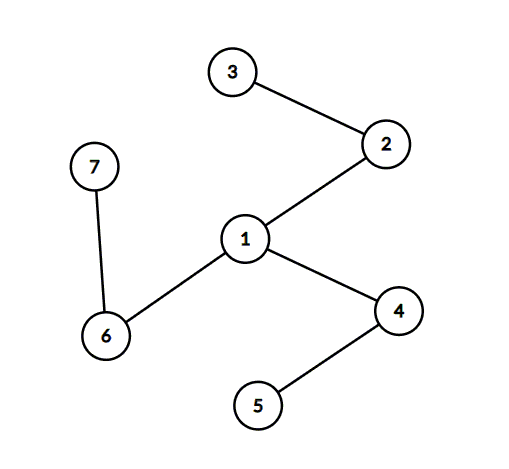시간 제한 메모리 제한 제출 정답 맞은 사람 정답 비율
1 초 512 MB 0 0 0 0.000%

## 문제

Berland government has decided to build a system of high speed railroads in the country. There are $n$ cities in Berland, numbered from $1$ to $n$. Some pairs of cities will be connected by a high speed railroad. Travelers will be able to move by train in both directions along each road.

High speed trains are the future of transport, so it is required to create such plan of railroad construction, that it is possible to get from any city to any other using railroads. To save money it was decided to build the minimal possible number of railroads to satisfy this criteria.

The government of the $i$-th city wants to support exactly $d_i$ trains. So the number of high speed railroads connecting the $i$-th city to others must be exactly $d_i$. Fortunately it turned out that $d_1 + d_2 + \ldots + d_n = 2n - 2$.

The government wants people to travel between cities using as few changes between trains as possible. You are asked to create such plan of railroads that the maximal number of changes needed to travel from a city to another was minimal.

## 입력

The first line of input contains an integer $n$ --- the number of cities in Berland ($2 \leq n \leq 200\,000$). The next line contains $n$ integers: $d_1, d_2, \ldots, d_n$ ($1 \leq d_i < n$). The number of cities connected to the $i$-th city must be $d_i$ for each $i$. It is guaranteed that $d_1 + d_2 + \ldots + d_n = 2n - 2$.

## 출력

Output $n-1$ lines --- the descriptions of the railroads in the optimal plan. Each line must contain two integers $s_i$ and $f_i$ --- cities to be connected by a railroad, $1 \leq s_i, f_i \leq n$, $s_i \neq f_i$.

The number of cities connected to the $i$-th city must be equal to $d_i$ for all $i$. The maximum number of train changes needed to travel from a city to another must be minimal possible. If there are several optimal plans, output any of them. It is guaranteed that there is at least one plan that satisfies all conditions.

## 예제 입력 1

7
3 2 1 2 1 2 1


## 예제 출력 1

1 2
2 3
1 4
4 5
1 6
6 7


## 예제 입력 2

4
1 2 2 1


## 예제 출력 2

1 2
2 3
3 4


## 힌트

The optimal answer for the first sample test is the following:It is possible to get from any city to any other using railroads. Each city has the required number of connected cities, for example the city $1$ is connected to three cities: $2$, $4$ and $6$ ($d_1=3$).

The maximum number of train changes needed to get from a city to another is for example for cities $3$ and $5$, $3$ transfers are needed. First you must travel from city $3$ to city $2$, then from city $2$ to city $1$, then from city $1$ to city $4$, and finally from city $4$ to city $5$. There is no plan that requires fewer changes for all pairs of cities.